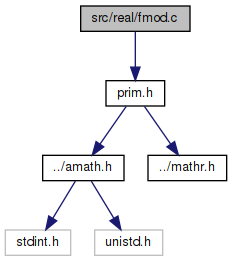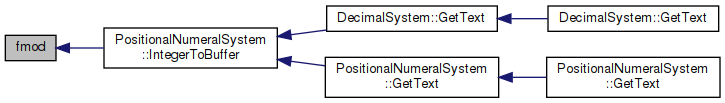amath  1.8.5 Simple command line calculator
fmod.c File Reference

Return x mod y in exact arithmetic. More...

`#include "prim.h"`
Include dependency graph for fmod.c:Go to the source code of this file.

## Functions

double fmod (double x, double y)
Return x mod y in exact arithmetic. More...

## Variables

static const double one = 1.0

static const double Zero []

## Detailed Description

Return x mod y in exact arithmetic.

Definition in file fmod.c.

## ◆ fmod()

 double fmod ( double x, double y )

Return x mod y in exact arithmetic.

Method: Shift and subtract

Definition at line 58 of file fmod.c.

References one, and Zero.

Referenced by PositionalNumeralSystem::IntegerToBuffer().

59 {
60  int32_t n, hx, hy, hz, ix, iy, sx, i;
61  uint32_t lx, ly, lz;
62
63  EXTRACT_WORDS(hx, lx, x);
64  EXTRACT_WORDS(hy, ly, y);
65  sx = hx & 0x80000000; /* sign of x */
66  hx ^= sx; /* |x| */
67  hy &= 0x7fffffff; /* |y| */
68
69  /* purge off exception values */
70  if ((hy | ly) == 0 || (hx >= 0x7ff00000) || /* y=0,or x not finite */
71  ((hy | ((ly | -ly) >> 31)) > 0x7ff00000)) /* or y is NaN */
72  return (x * y) / (x * y);
73  if (hx <= hy)
74  {
75  if ((hx < hy) || (lx < ly))
76  return x; /* |x|<|y| return x */
77  if (lx == ly)
78  return Zero[(uint32_t)sx >> 31]; /* |x|=|y| return x*0*/
79  }
80
81  /* determine ix = ilogb(x) */
82  if (hx < 0x00100000)
83  { /* subnormal x */
84  if (hx == 0)
85  {
86  for (ix = -1043, i = lx; i > 0; i <<= 1)
87  ix -= 1;
88  }
89  else
90  {
91  for (ix = -1022, i = (hx << 11); i > 0; i <<= 1)
92  ix -= 1;
93  }
94  }
95  else
96  ix = (hx >> 20) - 1023;
97
98  /* determine iy = ilogb(y) */
99  if (hy < 0x00100000)
100  { /* subnormal y */
101  if (hy == 0)
102  {
103  for (iy = -1043, i = ly; i > 0; i <<= 1)
104  iy -= 1;
105  }
106  else
107  {
108  for (iy = -1022, i = (hy << 11); i > 0; i <<= 1)
109  iy -= 1;
110  }
111  }
112  else
113  iy = (hy >> 20) - 1023;
114
115  /* set up {hx,lx}, {hy,ly} and align y to x */
116  if (ix >= -1022)
117  hx = 0x00100000 | (0x000fffff & hx);
118  else
119  { /* subnormal x, shift x to normal */
120  n = -1022 - ix;
121  if (n <= 31)
122  {
123  hx = (hx << n) | (lx >> (32 - n));
124  lx <<= n;
125  }
126  else
127  {
128  hx = lx << (n - 32);
129  lx = 0;
130  }
131  }
132  if (iy >= -1022)
133  hy = 0x00100000 | (0x000fffff & hy);
134  else
135  { /* subnormal y, shift y to normal */
136  n = -1022 - iy;
137  if (n <= 31)
138  {
139  hy = (hy << n) | (ly >> (32 - n));
140  ly <<= n;
141  }
142  else
143  {
144  hy = ly << (n - 32);
145  ly = 0;
146  }
147  }
148
149  /* fix point fmod */
150  n = ix - iy;
151  while (n--)
152  {
153  hz = hx - hy;
154  lz = lx - ly;
155  if (lx < ly)
156  hz -= 1;
157  if (hz < 0)
158  {
159  hx = hx + hx + (lx >> 31);
160  lx = lx + lx;
161  }
162  else
163  {
164  if ((hz | lz) == 0) /* return sign(x)*0 */
165  return Zero[(uint32_t)sx >> 31];
166  hx = hz + hz + (lz >> 31);
167  lx = lz + lz;
168  }
169  }
170  hz = hx - hy;
171  lz = lx - ly;
172  if (lx < ly)
173  hz -= 1;
174  if (hz >= 0)
175  {
176  hx = hz;
177  lx = lz;
178  }
179
180  /* convert back to floating value and restore the sign */
181  if ((hx | lx) == 0) /* return sign(x)*0 */
182  return Zero[(unsigned)sx >> 31];
183  while (hx < 0x00100000)
184  { /* normalize x */
185  hx = hx + hx + (lx >> 31);
186  lx = lx + lx;
187  iy -= 1;
188  }
189  if (iy >= -1022)
190  { /* normalize output */
191  hx = ((hx - 0x00100000) | ((iy + 1023) << 20));
192  INSERT_WORDS(x, hx | sx, lx);
193  }
194  else
195  { /* subnormal output */
196  n = -1022 - iy;
197  if (n <= 20)
198  {
199  lx = (lx >> n) | ((uint32_t)hx << (32 - n));
200  hx >>= n;
201  }
202  else if (n <= 31)
203  {
204  lx = (hx << (32 - n)) | (lx >> n);
205  hx = sx;
206  }
207  else
208  {
209  lx = hx >> (n - 32);
210  hx = sx;
211  }
212  INSERT_WORDS(x, hx | sx, lx);
213  x *= one; /* create necessary signal */
214  }
215  return x; /* exact output */
216 }
#define INSERT_WORDS(d, ix0, ix1)
Set a double from two 32 bit ints.
Definition: prim.h:187
static const double Zero[]
Definition: fmod.c:50
#define EXTRACT_WORDS(ix0, ix1, d)
Get two 32 bit ints from a double.
Definition: prim.h:156
static const double one
Definition: fmod.c:49
Here is the caller graph for this function:## ◆ one

 const double one = 1.0
static

Definition at line 49 of file fmod.c.

Referenced by fmod().

## ◆ Zero

 const double Zero[]
static
Initial value:
= {
0.0, -0.0,
}

Definition at line 50 of file fmod.c.

Referenced by fmod().